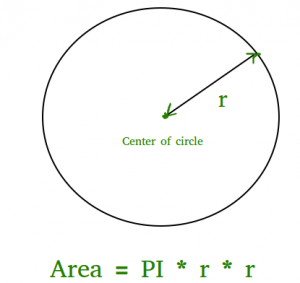# Program to find area of a circle

Given the radius of a circle, find the area of that circle.

The area of a circle can simply be evaluated using the following formula.where r is radius of circle and it maybe in float because value of pie is 3.14

Approach: Using the given radius, find the area using the above formula: (pi * r * r) and print the result in float.

## C++

 // C++ program to find area  // of circle  #include  const double pi = 3.14159265358979323846;  using namespace std;     // function to calculate the area of circle  float findArea(float r)  {      return (pi * r * r);  }  // driver code  int main()  {      float r, Area;      r = 5;         // function calling      Area = findArea(r);      // displaying the area      cout << "Area of Circle is :" << Area;      return 0;  }

## C

 // C program to find area  // of circle  #include  #include  #define PI 3.142     double findArea(int r)  {     return PI * pow(r, 2);  }     int main()  {      printf("Area is %f", findArea(5));      return 0;  }

## Java

 // Java program to find area  // of circle         class Test  {      static final double PI = Math.PI;                  static double findArea(int r)      {         return PI * Math.pow(r, 2);      }                  // Driver method      public static void main(String[] args)       {          System.out.println("Area is " +  findArea(5));      }  }

## Python3

 # Python3 program to find Area of a circle     def findArea(r):      PI = 3.142     return PI * (r*r);     # Driver method  print("Area is %.6f" % findArea(5));     # This code is contributed by Chinmoy Lenka

## C#

 // C# program to find area of circle  using System;     class GFG  {      static double PI = Math.PI;                 static double findArea(int r)      {      return PI * Math.Pow(r, 2);      }                 // Driver method      static void Main()       {          Console.Write("Area is " + findArea(5));      }  }     // This code is contributed by Sam007.

## PHP

 

## Javascript

 

Output

Area of Circle is :78.5398

Time Complexity: O(1)
Auxiliary Space: O(1), since no extra space has been taken.

Feeling lost in the world of random DSA topics, wasting time without progress? It's time for a change! Join our DSA course, where we'll guide you on an exciting journey to master DSA efficiently and on schedule.
Ready to dive in? Explore our Free Demo Content and join our DSA course, trusted by over 100,000 geeks!

Previous
Next